Home Practice
For learners and parents For teachers and schools
Textbooks
Full catalogue
Pricing SupportLog in

We think you are located in United States. Is this correct?

# End of chapter exercises

## End of chapter exercises

Textbook Exercise 21.8

Give one word/term for the following descriptions.

1. The shortest path from start to finish.

2. A physical quantity with magnitude and direction.

3. The quantity defined as a change in velocity over a time period.

4. The point from where you take measurements.

5. The distance covered in a time interval.

6. The velocity at a specific instant in time.

Solution not yet available

Choose an item from column B that match the description in column A. Write down only the letter next to the question number. You may use an item from column B more than once.

 Column A Column B a. The area under a velocity - time graph gradient b. The gradient of a velocity - time graph area c. The area under an acceleration - time graph velocity d. The gradient of a position - time graph displacement acceleration slope
Solution not yet available

Indicate whether the following statements are TRUE or FALSE. Write only “true” or “false”. If the statement is false, write down the correct statement.

1. A scalar is the displacement of an object over a time interval.

2. The position of an object is where it is located.

3. The sign of the velocity of an object tells us in which direction it is travelling.

4. The acceleration of an object is the change of its displacement over a period in time.

Solution not yet available

[SC 2003/11] A body accelerates uniformly from rest for $${t}_{0}$$ seconds after which it continues with a constant velocity. Which graph is the correct representation of the body's motion?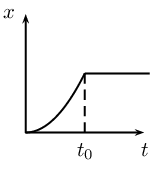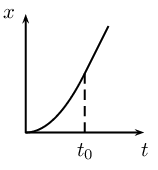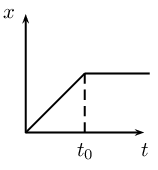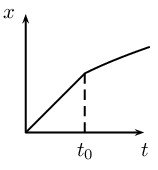(a) (b) (c) (d)
Solution not yet available

[SC 2003/11] The velocity-time graphs of two cars are represented by $$P$$ and $$Q$$ as shown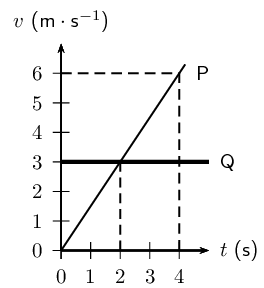The difference in the distance travelled by the two cars (in m) after $$\text{4}$$ $$\text{s}$$ is $$...$$

1. $$\text{12}$$

2. $$\text{6}$$

3. $$\text{2}$$

4. $$\text{0}$$

Solution not yet available

[IEB 2005/11 HG] The graph that follows shows how the speed of an athlete varies with time as he sprints for $$\text{100}$$ $$\text{m}$$.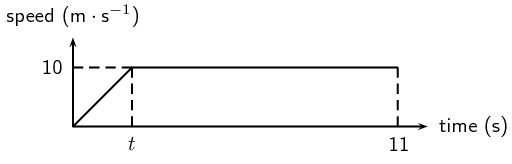Which of the following equations can be used to correctly determine the time t for which he accelerates?

1. $$100=\left(10\right)\left(11\right)-\frac{1}{2}\left(10\right)t$$

2. $$100=\left(10\right)\left(11\right)+\frac{1}{2}\left(10\right)t$$

3. $$100=10t+\frac{1}{2}\left(10\right){t}^{2}$$

4. $$100=\frac{1}{2}\left(0\right)t+\frac{1}{2}\left(10\right){t}^{2}$$

Solution not yet available

[SC 2002/03 HG1] In which one of the following cases will the distance covered and the magnitude of the displacement be the same?

1. A girl climbs a spiral staircase.

2. An athlete completes one lap in a race.

3. A raindrop falls in still air.

4. A passenger in a train travels from Cape Town to Johannesburg.

Solution not yet available

[SC 2003/11] A car, travelling at constant velocity, passes a stationary motor cycle at a traffic light. As the car overtakes the motorcycle, the motorcycle accelerates uniformly from rest for $$\text{10}$$ $$\text{s}$$. The following displacement-time graph represents the motions of both vehicles from the traffic light onwards.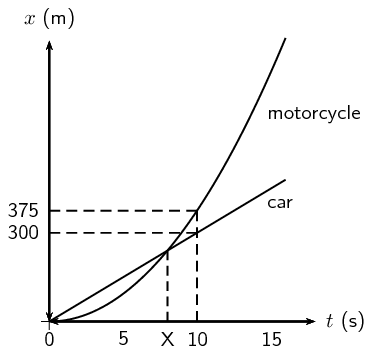1. Use the graph to find the magnitude of the constant velocity of the car.

2. Use the information from the graph to show by means of calculation that the magnitude of the acceleration of the motorcycle, for the first 10 s of its motion is $$\text{7,5}$$ $$\text{m·s^{-2}}$$.

3. Calculate how long (in seconds) it will take the motorcycle to catch up with the car (point $$X$$

4. How far behind the motorcycle will the car be after $$\text{15}$$ seconds?

Solution not yet available

[IEB 2005/11 HG] Which of the following statements is true of a body that accelerates uniformly?

1. Its rate of change of position with time remains constant.

2. Its position changes by the same amount in equal time intervals.

3. Its velocity increases by increasing amounts in equal time intervals.

4. Its rate of change of velocity with time remains constant.

Solution not yet available

[IEB 2003/11 HG1] The velocity-time graph for a car moving along a straight horizontal road is shown below.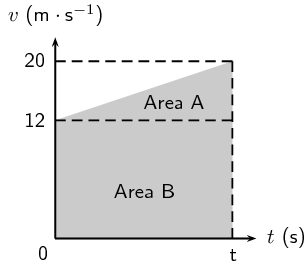Which of the following expressions gives the magnitude of the average velocity of the car?

1. $$\frac{\text{Area } A}{t}$$

2. $$\frac{\text{Area } A + \text{ Area } B}{t}$$

3. $$\frac{\text{Area } B}{t}$$

4. $$\frac{\text{Area } A - \text{ Area }B}{t}$$

Solution not yet available

[SC 2002/11 SG] A car is driven at 25 $$\text{m·s^{-1}}$$ in a municipal area. When the driver sees a traffic officer at a speed trap, he realises he is travelling too fast. He immediately applies the brakes of the car while still $$\text{100}$$ $$\text{m}$$ away from the speed trap.

1. Calculate the magnitude of the minimum acceleration which the car must have to avoid exceeding the speed limit, if the municipal speed limit is $$\text{16,6}$$ $$\text{m·s^{-1}}$$.

2. Calculate the time from the instant the driver applied the brakes until he reaches the speed trap. Assume that the car's velocity, when reaching the trap, is $$\text{16,6}$$ $$\text{m·s^{-1}}$$.

Solution not yet available

A traffic officer is watching his speed trap equipment at the bottom of a valley. He can see cars as they enter the valley $$\text{1}$$ $$\text{km}$$ to his left until they leave the valley $$\text{1}$$ $$\text{km}$$ to his right. Nelson is recording the times of cars entering and leaving the valley for a school project. Nelson notices a white Toyota enter the valley at $$\text{11}$$:$$\text{01}$$:$$\text{30}$$ and leave the valley at $$\text{11}$$:$$\text{02}$$:$$\text{42}$$. Afterwards, Nelson hears that the traffic officer recorded the Toyota doing $$\text{140}$$ $$\text{km·h^{-1}}$$.

1. What was the time interval ($$\Delta t$$

2. What was the average speed of the Toyota?

3. Convert this speed to $$\text{km·h^{-1}}$$

4. Discuss whether the Toyota could have been travelling at $$\text{140}$$ $$\text{km·h^{-1}}$$ at the bottom of the valley.

5. Discuss the differences between the instantaneous speed (as measured by the speed trap) and average speed (as measured by Nelson).

Solution not yet available

[IEB 2003/11HG] A velocity-time graph for a ball rolling along a track is shown below. The graph has been divided up into $$\text{3}$$ sections, A, B and C for easy reference. (Disregard any effects of friction.)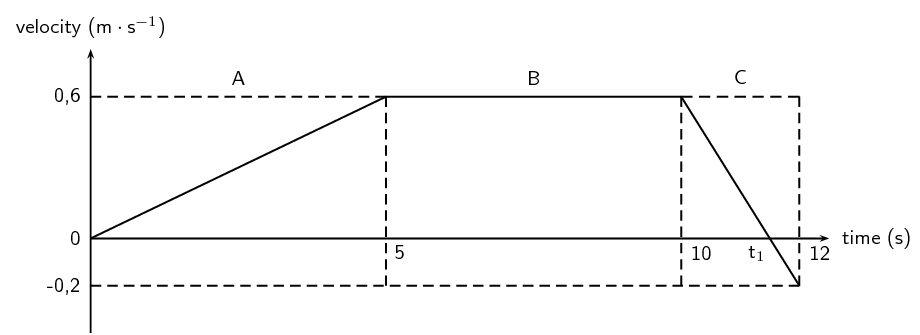1. Use the graph to determine the following:

1. the speed $$\text{5}$$ $$\text{s}$$ after the start

2. the distance travelled in Section A

3. the acceleration in Section C

2. At time $${t}_{1}$$$${t}_{1}$$

3. Sketch a displacement-time graph for the motion of the ball for these $$\text{12}$$ $$\text{s}$$. (You do not need to calculate the actual values of the displacement for each time interval, but do pay attention to the general shape of this graph during each time interval.)

Solution not yet available

In towns and cities, the speed limit is $$\text{60}$$ $$\text{km·h^{-1}}$$. The length of the average car is $$\text{3,5}$$ $$\text{m}$$, and the width of the average car is $$\text{2}$$ $$\text{m}$$. In order to cross the road, you need to be able to walk further than the width of a car, before that car reaches you. To cross safely, you should be able to walk at least $$\text{2}$$ $$\text{m}$$ further than the width of the car ($$\text{4}$$ $$\text{m}$$ in total), before the car reaches you.

1. If your walking speed is $$\text{4}$$ $$\text{km·h^{-1}}$$, what is your walking speed in $$\text{m·s^{-1}}$$?

2. How long does it take you to walk a distance equal to the width of the average car?

3. What is the speed in $$\text{m·s^{-1}}$$ of a car travelling at the speed limit in a town?

4. How many metres does a car travelling at the speed limit travel, in the same time that it takes you to walk a distance equal to the width of car?

5. Why is the answer to the previous question important?

6. If you see a car driving toward you, and it is $$\text{28}$$ $$\text{m}$$ away (the same as the length of $$\text{8}$$ cars), is it safe to walk across the road?

7. How far away must a car be, before you think it might be safe to cross? How many car-lengths is this distance?

Solution not yet available

A bus on a straight road starts from rest at a bus stop and accelerates at $$\text{2}$$ $$\text{m·s^{-2}}$$ until it reaches a speed of $$\text{20}$$ $$\text{m·s^{-1}}$$. Then the bus travels for $$\text{20}$$ $$\text{s}$$ at a constant speed until the driver sees the next bus stop in the distance. The driver applies the brakes, stopping the bus in a uniform manner in $$\text{5}$$ $$\text{s}$$.

1. How long does the bus take to travel from the first bus stop to the second bus stop?

2. What is the average velocity of the bus during the trip?

Solution not yet available